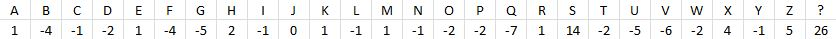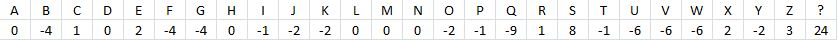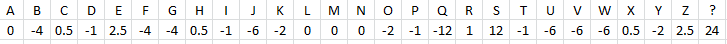# No Tile Left Behind Series: Single Tile Values

Leave is one of the first strategic concepts that most Scrabble players learn. While leaves are mostly intuitive, many of the nuances escape amateur players.  Failing to understand these nuances will result in small errors that will quickly add up.

To start, let’s ask a simple question: What is the worth of a leave?

A leave’s worth is the number of points you are willing to give up to keep those tiles. The worth of a specific leave is determined by its ability to score on subsequent turns.  Bingo leaves are usually based on their propensity to form a bingo (making its value more polarized) while scoring leaves usually score less on average, but have a more consistent propensity to score well.

At this point, we can now ask the most fundamental questions in all of Scrabble: What is the worth of each individual tile?

# Tile Values

The worth of each tile (disregarding its contribution to vowel-consonant ratio, duplication, etc.) is called its tile value.  Once you understand the worth of each individual tile, it is much easier to develop a base approximation for the worth of any leave by simply summing the worth of each individual tile.

However, this isn’t such an easy question to answer.  While it may be obvious that the S is a good tile and the Q is a bad tile, it is not so obvious to what degree.  Furthermore, other tiles are not so immediately obvious.  Is the Y a good tile?  The P?  The T?  Using intuition, it’s really hard to tell.

For this reason, we turn to computers.  The first serious approach that people used was statistical analysis.  After some deliberation, the folllowing approach was implemented:

1.  Create a computer that would always make the highest scoring play.

2.  Run millions of random racks (millions) making the highest scoring play on each turn.

3.  Record the tiles on each rack and the corresponding number of points that each rack scored.

4.  Run a statistical correlation to find the average number of points scored whenever each tile was on your rack.

5.  Find the difference between the above value and the average score per turn.

6.  Once these numbers were produced, create a computer that would make the play that maximized score plus the sum of tiles.

7.  Repeat steps 2-5.

As a result, we got a table that looked something like this:At first, the table was lauded as a huge breakthrough in Scrabble theory.  It allowed people a precise mathematical representation of the average value of every tile.  This was great: finally Scrabble had a way to compare points and leave!

However, over time, theorists developed reservations.  While this was a good approximation of how each tile corresponds to the number of points you’d score each turn, this is not indicative of a leave.  A leave is the worth of those tiles contingent on those tiles not being a part of the best play.

For example, let’s say you have the Q on your rack.  If QI or a QU play is available, it is very likely this will be the best play.  When these options are not available, the Q becomes a worse tile.  And if there is no good Q spot now, there probably won’t be a good Q spot next turn.  This means very few 50 point Q and X plays, lucrative S hooks, etc.

In short, the Q is a worse tile when part of a leave compared to when it is first drawn.  The same logic holds for other tiles as well.  Because of this, a new, more sophisticated chart was created:  Whenever a tile is part of a leave, the value that should be calculated is the worth of a tile given that it is not used in the best play.  As a result, the following chart was created:Even still, there are some adjustments that need to be made. These values still do not account for defense, vowel-consonant ratio (yes, it matters even with one tile), synergy, duplication, or the ability to setup or make future plays with a given tile. Since the tiles above are dependent upon a one-ply iteration heuristic, it is impossible to account for these using the above heuristic: you must either adjust the heuristic drastically (which we currently cannot do in an efficient matter while obtaining enough data) or use simulations and make the adjustment manually. Below is an example of that manual adjustment, using largely intuition and corroborated by computer simulation:This results in the tile values that we have today.